# Debugging¶

## Visualising the system¶

It is sometimes useful when debugging a problem to see dolfin-adjoint’s interpretation of your forward system, and the other models it derives from that. The `visualise` function visualises the system as a graph using TensorFlow. To do this add

```tape = get_working_tape()
tape.visualise()
```

This will create a log directory with TensorFlow event files. We can then run TensorBoard, which comes bundled with TensorFlow, and point it to this log directory:

```tensorboard --logdir=log
```

By default the log directory is a directory `log` in the current working directory. This can be changed by specifying a directory in the `logdir` keyword argument to `visualise`.

Finally, we can open http://localhost:6006/ in a web browser to view the graph. To demonstrate, let us use a simplified version of our old Burgers’ equation example:

```from dolfin import *

n = 30
mesh = UnitSquareMesh(n, n)
V = VectorFunctionSpace(mesh, "CG", 2)

u = project(Expression(("sin(2*pi*x)", "cos(2*pi*x)"), degree=2),  V)
u_next = Function(V)
v = TestFunction(V)

nu = Constant(0.0001)

timestep = Constant(0.01)

F = (inner((u_next - u)/timestep, v)

bc = DirichletBC(V, (0.0, 0.0), "on_boundary")

solve(F == 0, u_next, bc)

tape = get_working_tape()
tape.visualise()
```

Here we solve the equation for only one timestep.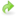Download the code to find graph.

Running TensorBoard and opening http://localhost:6006/ in our web browser shows us the following graph: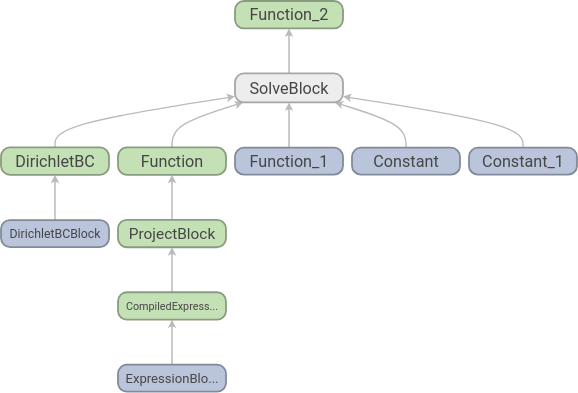Each node corresponds to an elementary operation, and we see that the structure is what we should expect: two functions, two constants and a set of boundary conditions go into an equation and we get one function out. To increase readability further we can add names to the functions:

```from dolfin import *

n = 30
mesh = UnitSquareMesh(n, n)
V = VectorFunctionSpace(mesh, "CG", 2)

u = project(Expression(("sin(2*pi*x)", "cos(2*pi*x)"), degree=2),  V)
u_next = Function(V, name="u_next")
v = TestFunction(V)

nu = Constant(0.0001, name="nu")

timestep = Constant(0.01, name="dt")

F = (inner((u_next - u)/timestep, v)

bc = DirichletBC(V, (0.0, 0.0), "on_boundary")

solve(F == 0, u_next, bc)

tape = get_working_tape()
tape.visualise()
```

The resulting graph is the following: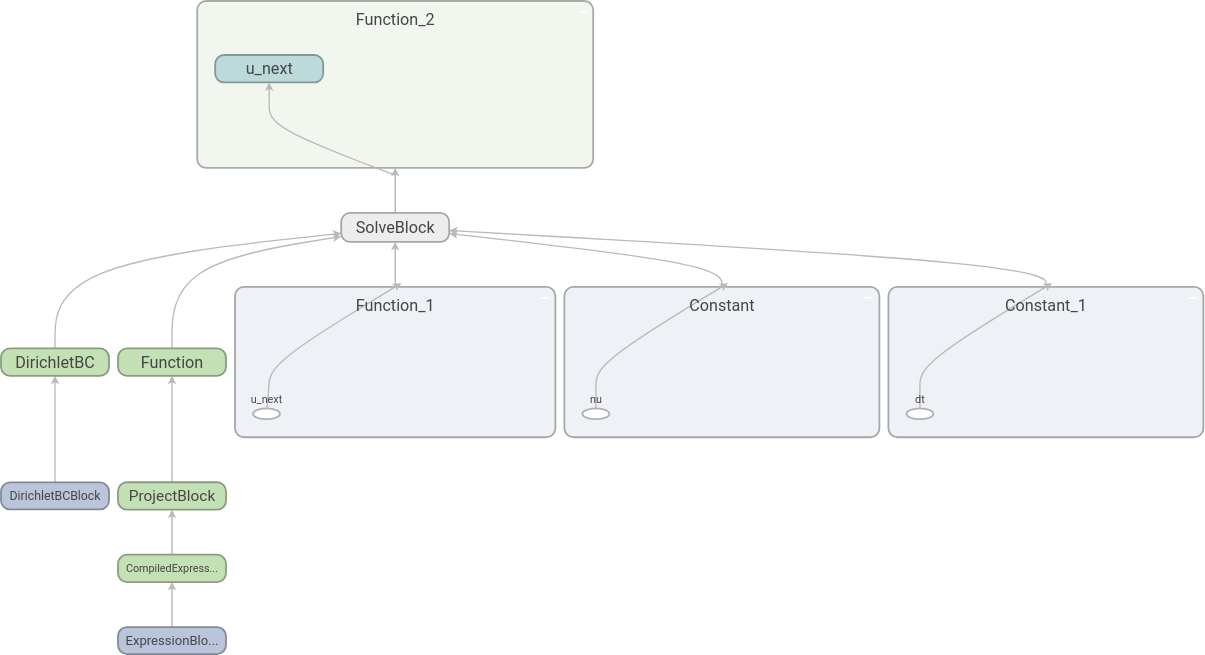Here we have expanded some of the nodes by double-clicking on them. We see that it is indeed `u_next` that is found by the equation solve.Download the code to find the graph with names.

If we have a time loop, the graph can easily become large and difficult to read. The `name_scope` factory function can be used to make this easier. To demonstrate this, we add a time loop to our example above:

```from dolfin import *

n = 30
mesh = UnitSquareMesh(n, n)
V = VectorFunctionSpace(mesh, "CG", 2)

u = project(Expression(("sin(2*pi*x)", "cos(2*pi*x)"), degree=2),  V)
u_next = Function(V, name="u_next")
v = TestFunction(V)

nu = Constant(0.0001, name="nu")

timestep = Constant(0.01, name="dt")

F = (inner((u_next - u)/timestep, v)

bc = DirichletBC(V, (0.0, 0.0), "on_boundary")

tape = get_working_tape()

t = 0.0
end = 0.05
while (t <= end):
with tape.name_scope("Timestep"):
solve(F == 0, u_next, bc)
u.assign(u_next)
t += float(timestep)

tape.visualise()
```

Here, we should note the line after we enter the `while` loop. In this line we use a `with` statement together with `name_scope` and a name for the scope. This will ensure that all nodes corresponding to one time step will be added to its own scope. After the time loop, we call `visualise` and get the following graph: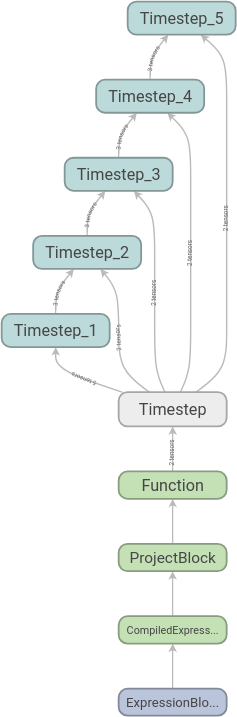We can then expand the scopes as we like. Here we have expanded the first time step: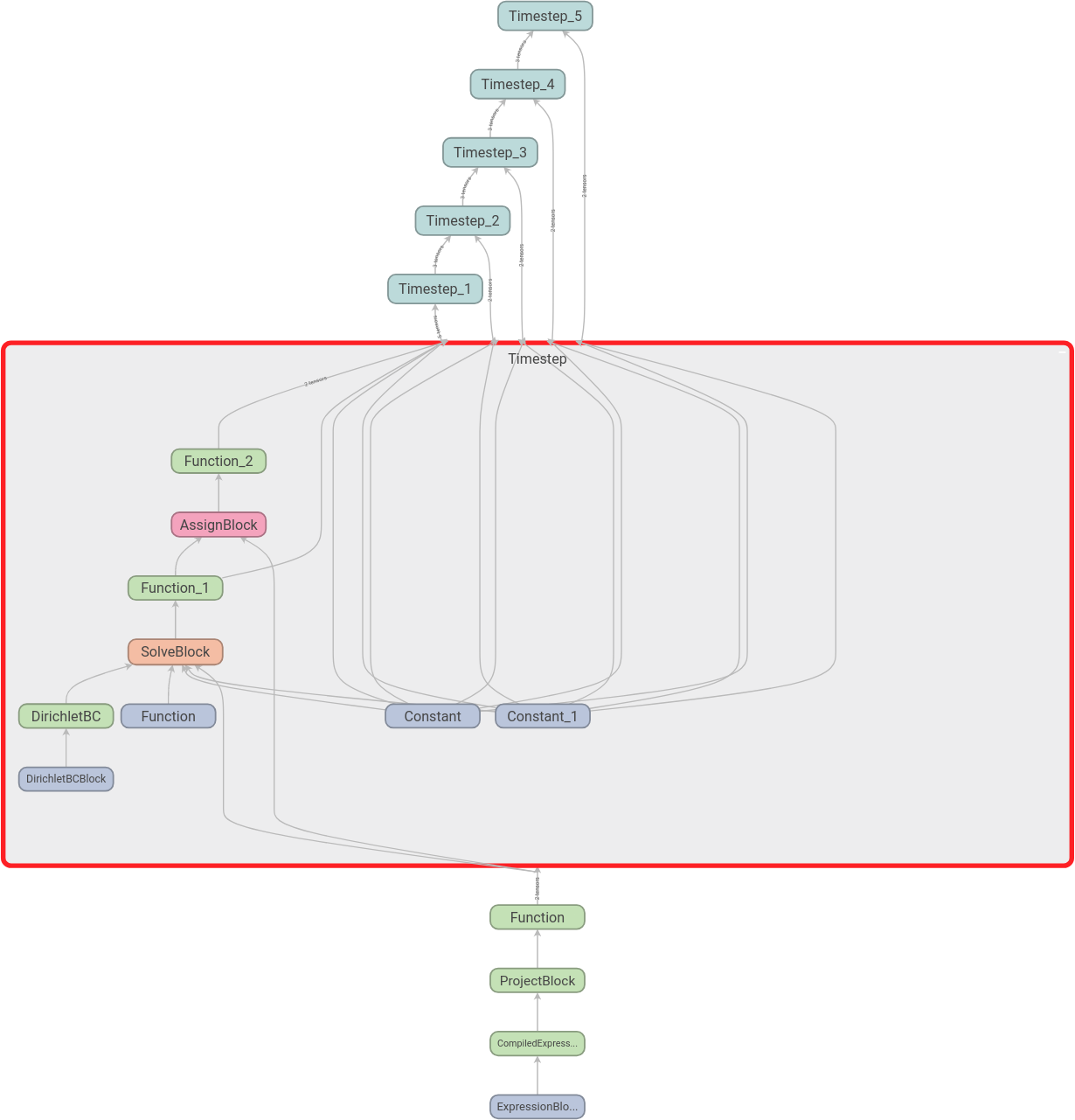Download the code to find the graph with name scopes.

In the next section we discuss parallelisation.